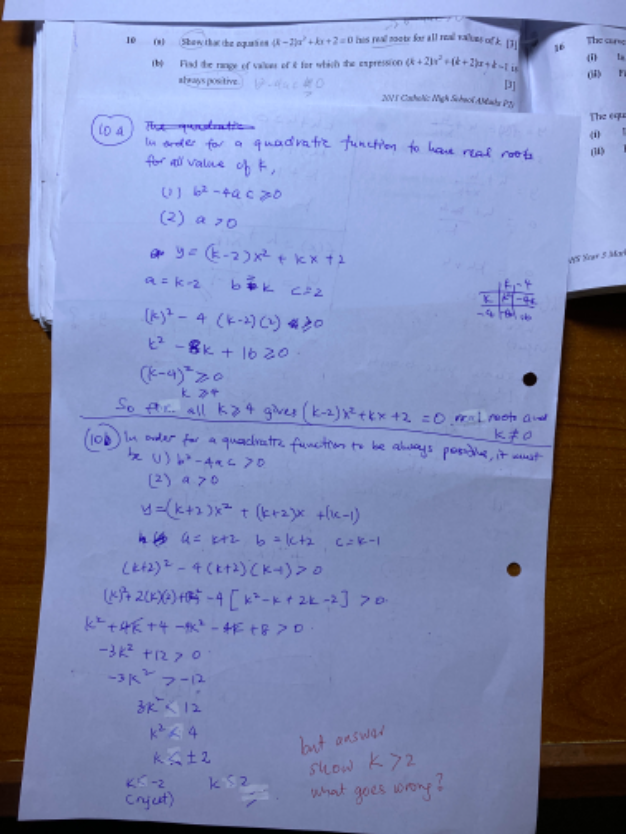# QuestionKindly correct my 10a and why 10b answer k shows different sign?

The concept of D is incorrect.   To check for positive or negative definite quadratic function, always check D < 0.

Quadratic function is always negative if:

1. D < 0
2. a < 0

Quadratic function is always positive if:

1. D < 0
2. a > 0

10 (b) is using the concept in green.

So there is a sign difference in your working only.

From this step:

-3k2 + 12 < 0     (multiply by -1/3)

k2 – 4 > 0

(k + 2) (k – 2)  > 0                           ———–|———–|———–

-2                2

For the expression to be > 0,   the solution should be found in the red part on the number line, meaning    k < -2   or  k > 2

Since a > 0 => k+ 2 > 0  => k > -2,  so reject k < -2

The solution for k is k > 2

2 Replies 1 Like ✔Accepted Answer

So is my answer for 10a is ok?

To check for real roots,  only need to determine if D  > 0   so condition 2 to delete because not necessary for checking real roots(that is only required for checking positive definite)

Based on your working of D,

k2 – 8k + 16 = (k-4)2

This means that for all values of k,  (k-4)2 >  0

Hence (k-2)x2 + kx + 2 = 0 has real roots for all values of k.

0 Replies 1 Like

yes, i am really confused sometime, but thanks again for your this explanation. 🙂 cheers.

0 Replies 0 Likes